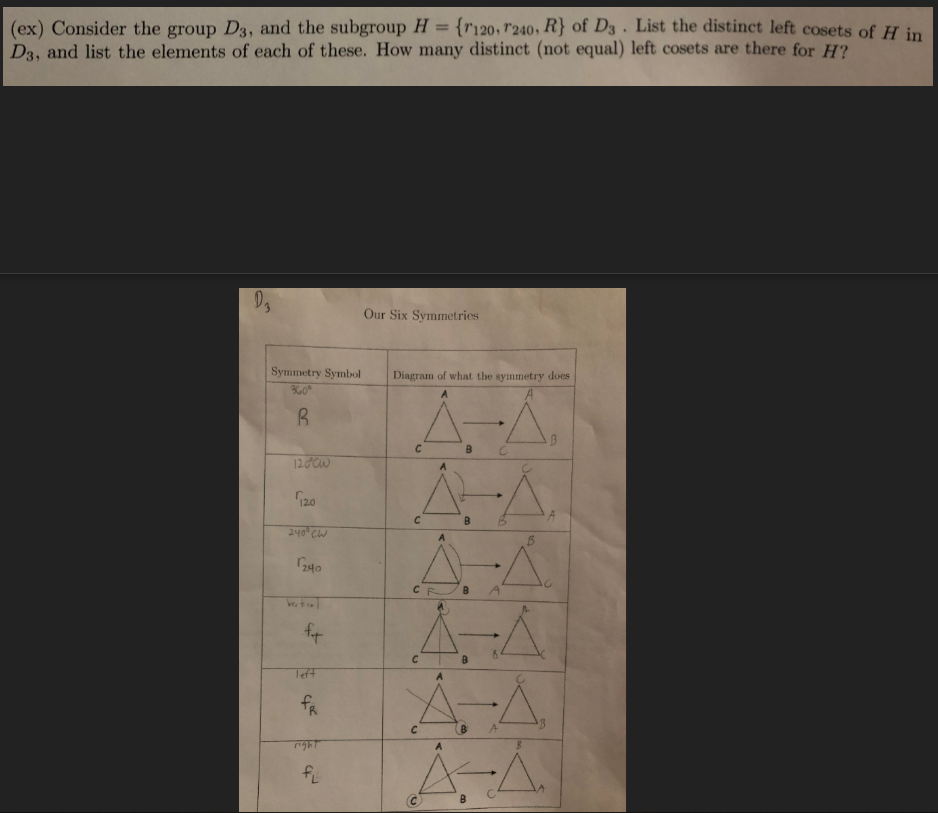# (ex) Consider the group Ds, and the subgroup H (r120,r240. R) of Ds List the distinct left cosets of H inD3, and list the elements of each of these. How many distinct (not equal) left cosets are there for H?Our Six SymmetriesSymmetry Symbol Diagram of what the synmetry does12024oB A

Question
18 views

Abstract Algebra.

D3 is given in the picture as well as the problem.help_outlineImage Transcriptionclose(ex) Consider the group Ds, and the subgroup H (r120,r240. R) of Ds List the distinct left cosets of H in D3, and list the elements of each of these. How many distinct (not equal) left cosets are there for H? Our Six Symmetries Symmetry Symbol Diagram of what the synmetry does 120 24o B A fullscreen
check_circle

Step 1
Step 2

The coset RK can be listed as follows.

Step 3

The coset r120K can be listed a...

### Want to see the full answer?

See Solution

#### Want to see this answer and more?

Solutions are written by subject experts who are available 24/7. Questions are typically answered within 1 hour.*

See Solution
*Response times may vary by subject and question.
Tagged in

### Math